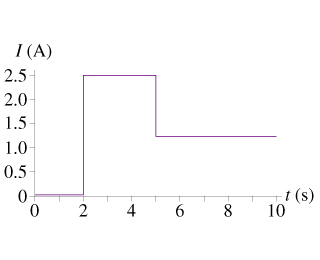# Problem: The graph in the figure shows the current through a 1.0ohm resistor as a function of time.How much charge flowed through the resistor during the 10 s interval shown? What was the total energy dissipated by the resistor during this time?

###### FREE Expert Solution

(a)

Charge:

$\overline{){\mathbf{Q}}{\mathbf{=}}{\mathbf{i}}{\mathbf{t}}}$

1A = 1C/s

i•t is the area under the curve.

There are three areas: 0-2s, 2-5s, and 5-10s.

95% (118 ratings)###### Problem Details

The graph in the figure shows the current through a 1.0ohm resistor as a function of time.How much charge flowed through the resistor during the 10 s interval shown?

What was the total energy dissipated by the resistor during this time?